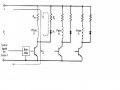# How to solve this problem?

#### lihker

Joined Dec 3, 2008
3
The unipolar drive circuit of a three phase variable reluctance stepper motor used for positioning a valve is shown inThe motor has the following parameters :
Winding resistance (RW) = 0.6 Ω Winding inductance (LW)=0.24 mH.
Rated current = 5 A he stepping rate = 450 steps/sec

Design the drive circuit such that the electrical time constant is 1.5 ms at turn-on and 0.8 ms at turn-off.

We want to find out the R(ext) value using T (on)=Lw/Rw+R(ext)
But after i calculate R(ext)=-0.44Ω(resistance do not have negative value) something wrong!!

somebody help me solve thank

#### mik3

Joined Feb 4, 2008
4,843
Are you sure about the values?